# If the 3

If the temperature of a city drops 2° everyday and increases by 3° on every 5 days, how long does it take to drop to -15° from temperature +5°?

n =  20 d

### Step-by-step explanation: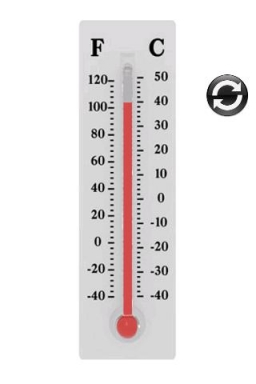Did you find an error or inaccuracy? Feel free to write us. Thank you!## Related math problems and questions:

• The temperature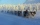The temperature is 0 degrees. It drops 5 degrees after 1 hour and then drops 3 degrees more the next hour. What is the temperature after 6 hours?
• Temperature rise and fallOn Friday, the temperature was 82°F. The temperature changed by –2°F on Saturday, and then it changed by 5°F on Sunday. What was the temperature on Sunday? How did the temperature change? Note we consider the mathematical problem formulated in this way to
• The temperature 10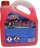The temperature in a freezer is -15°C, and it increases by 3°C.
• Temperature differenceLibya's highest temperature was recorded at 58 degrees Celsius, and the lowest was recorded at -88 degrees Celsius. What is the temperature difference?
• The temperature 5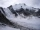The temperature at 4pm was 53° Fahrenheit the temperature dropped 3° each hour from 4pm to 9pm which equation below accurately represents the temperature at 9pm
• Temperature variationsToday's temperature was 80 degrees, and then the temperature dropped 10 degrees. Then it dropped 15 degrees again, then the next day, the temperature went up 2 degrees. What would the temperature be?
• Now isNow is 5°C morning. What will the temperature be if i. It rises by 12°C ii. It falls by 15°C
• RoxvilleThe temperature in Roxville decreased 15 degree in five hours. What was the average decrease in temperature per hour? Write you answer as an integer.
• Temperature 5Temperature outside starts at 0 Fahrenheit. Over time the temperature changes at a rate of -0.6 Fahrenheit per hour. How long will it take for the temperature to reach -4.5 Fahrenheit?
• Temperature changeThe mean temperature change is -3.2°F per day for five days. What is the total change over the five day period?
• Division by zero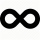Fraction 5 by 2. if 3 is added to numerator and 2 is subtracted from the denominator then the new fraction is:
• On SundayOn Sunday the temperature reached a high of 38° above 0. That night it dropped to 23° below 0. What is the difference between the high and low temperatures for Sunday?
• To improper fractionChange mixed number to improper fraction a) 1 2/15 b) -2 15/17
• Difference in temperaturesThe temperature in the number line show minus 15 degree celsius and 8 degree celsius. What is the difference between the temperatures?
• 5 2/55 2/5 hours a week  mathematics,  3 3/4 hours a week   Natural sciences, 4 3/8 hours a week  Technology . how many hours does  he spend on social sciences if he spend 17 1/2 hours a week for the four subject?
• The temperature 4The temperature was freezing, then it doubled, then it dropped 10 degrees, and then it increased by 40 degrees. The temperature is now 16 degrees. What was the starting temperature?
• Alice 2Alice is swimming 2.5 hours every Saturday and 3.5 hours every Sunday. How many hours does she swim in 4 weeks?